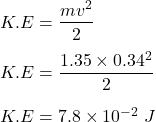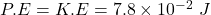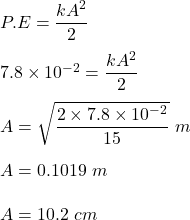## A 1.35 kgkg block is attached to a spring with spring constant 15.0 N/mN/m . While the block is sitting at rest, a student hits it with a ha

Question

A 1.35 kgkg block is attached to a spring with spring constant 15.0 N/mN/m . While the block is sitting at rest, a student hits it with a hammer and almost instantaneously gives it a speed of 34.0 cm/scm/s . What are

in progress 0
3 months 2021-07-29T14:21:51+00:00 1 Answers 4 views 0

1. Given :

Mass of block , m = 1.35 kg .

Speed constant , k = 15 N/m .

Speed at centre , v = 34 cm/s = 0.34 m/s .

To Find :

The amplitude of oscillation .

Solution :

Now , we know total energy is conserved in SHM .

So , K.E at zero displacement :Now , this K.E is equal to maximum P.E :.Therefore , the amplitude is 10.2 cm .

Hence , this is the required solution .